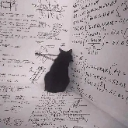2020-7-4 17:06
2630

# Galgame汉化中的逆向（三）：自定义字库分析

## 0x0 前言## 0x1 观察## 0x3 字库分析

`font48.xtx`字库编码为`GRAY4`，每个字符为48X48，共8192个字符。 block为48字节，每个block含4个坐标。

```block_size = 48
intensity_map = (
0b00000000, 0b00010001, 0b00100010, 0b00110011,
0b01000100, 0b01010101, 0b01100110, 0b01110111,
0b10001000, 0b10011001, 0b10101010, 0b10111011,
0b11001100, 0b11011101, 0b11101110, 0b11111111)

def get_x(i, width, level):
# level means nesting times
v1 = (level >> 2) + (level >> 1 >> (level >> 2))
v2 = i << v1
# 8X8 3f,
v3 = (v2 & 0x3F) + ((v2 >> 2) & 0x1C0) + ((v2 >> 3) & 0x1FFFFE00)
return ((((level << 3) - 1) & ((v3 >> 1) ^ ((v3 ^ (v3 >> 1)) & 0xF))) >> v1)+  ((((((v2 >> 6) & 0xFF) + ((v3 >> (v1 + 5)) & 0xFE)) & 3)
+ (((v3 >> (v1 + 7)) % (((width + 31)) >> 5)) << 2)) << 3)

def get_y(i, width, level):
v1 = (level >> 2) + (level >> 1 >> (level >> 2))
v2 = i << v1
v3 = (v2 & 0x3F) + ((v2 >> 2) & 0x1C0) + ((v2 >> 3) & 0x1FFFFE00)
return ((v3 >> 4) & 1) + ((((v3 & ((level << 6) - 1) & -0x20)
+ ((((v2 & 0x3F)
+ ((v2 >> 2) & 0xC0)) & 0xF) << 1)) >> (v1 + 3)) & -2) + ((((v2 >> 10) & 2) + ((v3 >> (v1 + 6)) & 1)
+ (((v3 >> (v1 + 7)) // ((width + 31) >> 5)) << 2)) << 3)

def xtx_tex12gray(data, height, width, aligned_height, aligned_width):
gray = np.zeros([width*2, height*2], dtype=np.uint8) # exchange width height

print("%dX%d xtx, %d bytes-> %dX%d Gray"%(width, height, len(data), height*2, width*2 ))
for i in range(height*width):
abs_x = get_x(i, width, 2)
abs_y = get_y(i, width, 2)
if abs_y >= height or abs_x >= width:
continue

# each 2 byte containes 4 gray pixel
idx = i * 2
block_x = (abs_x // block_size) * block_size
block_y = (abs_y // block_size) * block_size
x = abs_x % block_size
y = abs_y % block_size
target_y = block_y + y
target_x1 = block_x * 4 + x; # each block(48X48) has 4 cordinate
target_x2 = block_x * 4 + x + block_size;
target_x3 = block_x * 4 + x + block_size * 2;
target_x4 = block_x * 4 + x + block_size * 3;
gray[target_y][target_x1] = intensity_map[data[idx] >> 4]
gray[target_y][target_x2] = intensity_map[data[idx] & 0xf]
gray[target_y][target_x3] = intensity_map[data[idx+1] >> 4]
gray[target_y][target_x4] = intensity_map[data[idx+1] & 0xf]
return gray
```

```def showtable():
width = 48
height = 96
lines = []
for i in range(height*2):
lines.append(width*2*)
for i in range(width * height):
x = get_x(i, width, 2)
y = get_y(i, width, 2)
lines[y][x] = str(i)
print(i, y ,x)
with open('position.csv', 'w', newline='') as fp:
f_csv = csv.writer(fp)
f_csv.writerows(lines)
```

（怪不的位运算那么一坨乱七八糟的，所以才说禁止套娃呀#滑稽）## 0x4 重建字库

(1) 建立简体字库的png图片，
(2) 修改fontmap或者将gb2312编码映射到sjis上，生成映射后的码表（.tbl）
(3) 将png转换为该游戏字库格式

（1）建立图片这个方法太多了，要求是每个字大小48X48，图大小6144X3072。
最简单的方法是用PIL的ImageFont打印，其中大小px要转换为pt。
（2）此游戏的fontmap我没找到，严重怀疑游戏是通过计算来得到的图中位置。
sjis编码的范围是程序里写死的，要改动涉及到的地方太多太麻烦。
本游戏的字库基本上是完整的sjis字库，从0x8140开始每个sjis字符包括不可打印字符都有（除了末尾的部分字符），
因此直接把gb2312按顺序映射到sjis编码即可，新建字符顺序是sjis但字符是简体字。
(3) 这部解包和封包差不多，只要把src和dst方向对调就行（当然宽高大小之类的参数要微调一下）

https://github.com/YuriSizuku/GalgameReverse/

## 0x5 后记

Galgame汉化本身也是一种有意思的逆向，但是相对来说讨论的不多,打赏次数 1 金额 + 1.00天水姜伯约 1 2020/07/13 感谢分享～mb_hbzbnhpp2020-7-5 14:36
2
1hzqst3 2020-7-5 21:37
3
04
05
0coneco2 2020-7-19 19:02
6
0